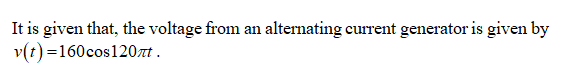# The voltage from an alternating current generator is given by V(t)=160cos120πt,where t is measured in seconds. Find the time t when the voltage first equals 80.step by step

Question
10 views

The voltage from an alternating current generator is given by V(t)=160cos120πt,where t is measured in seconds. Find the time t when the voltage first equals 80.

step by step

check_circle

Step 1Step 2

Obtain the time t when the voltage is 80,

Step 3

On further simpli...

### Want to see the full answer?

See Solution

#### Want to see this answer and more?

Solutions are written by subject experts who are available 24/7. Questions are typically answered within 1 hour.*

See Solution
*Response times may vary by subject and question.
Tagged in

### Other